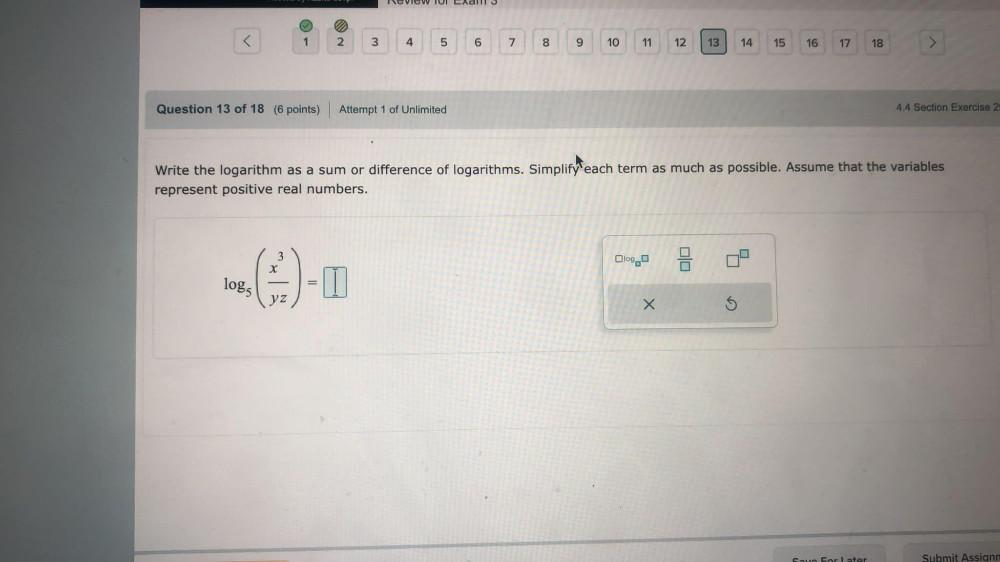Question:

# Write the logarithm as a sum or difference of logarithms. Simplify each term as much as possible. Assume that the variables represent positive real numbers. log₅ (x³/yz).Write the logarithm as a sum or difference of logarithms. Simplify each term as much as possible. Assume that the variables represent positive real numbers. log₅ (x³/yz).